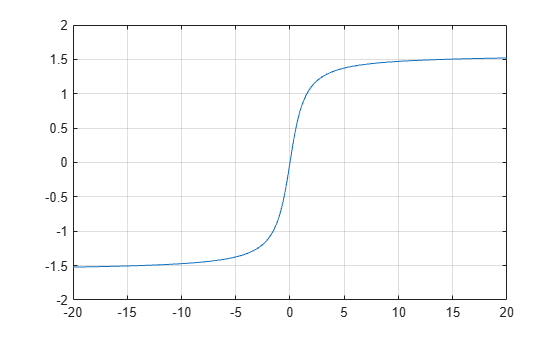atan

Description

example

Y = atan(X) returns the Inverse Tangent (tan-1) of the elements of X in radians. The function accepts both real and complex inputs.

• For real values of X, atan(X) returns values in the interval [-π/2, π/2].

• For complex values of X, atan(X) returns complex values.

Examples

collapse all

Find the inverse tangent of a value.

atan(0.8)
ans = 0.6747

Find the inverse tangent of the elements of vector x. The atan function acts on x element-wise.

x = [0.5i 1+3i -2.2+i];
Y = atan(x)
Y = 1×3 complex

0.0000 + 0.5493i   1.4615 + 0.3059i  -1.2019 + 0.1506i

Plot the inverse tangent function over the interval $-20\le x\le 20$.

x = -20:0.01:20;
plot(x,atan(x))
grid onInput Arguments

collapse all

Tangent of angle, specified as a scalar, vector, matrix, or multidimensional array. The atan operation is element-wise when X is nonscalar.

Data Types: single | double
Complex Number Support: Yes

collapse all

Inverse Tangent

The inverse tangent is defined as

${\mathrm{tan}}^{-1}\left(z\right)=\frac{i}{2}\mathrm{log}\left(\frac{i+z}{i-z}\right).$